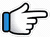# Dilbar Dilbar – Keyboard Notes – Hosh Na Khabar Hai## Dilbar Dilbar – Hosh Na Khabar Hai Keyboard Notes

For Dilbar Dilbar Notes as Western or Indian Notations, Click –

Western – CDE FGAB

Indian – srg mpdn

Hindi – सारेग मपधनि

## Keyboard Notes for Dilbar Dilbar – Indian Notation (srg mpdn)

CDE FGAB

सारेग मपधनि

Starting Music

Starting music notes
are given after the song notations.

Notes for first 6 lines of
part 1 and 2 are same and they are –

dd   r*r*   r*~  s*n  s* n~

### Part 1

suroor hai

Asar tera ye zaroor hai
Teri nazar ka kasoor hai

Dilbar dilbar

1. Chadha jo mujhpe suroor hai

dd             r*r*        r*~

s*n      s* nd~
suroo   r_ hai

2. Asar tera ye zaroor hai

dd      r*r*    r*~
Asar   tera  yeh

s*n      s* nd~
zaroo   r_ hai

3. Teri nazar ka kasoor hai, Dilbar dilbar

dd      r*r*        r*~
Teri    nazar     ka

s*n      s*  nd
kasoo  r_ hai

pd       pd~
Dilbar  dilbar
Or
p*d*    p*d*~

### Part 2

Aa pass aa
tu kyu door hai

Ye ishq ka jo fitoor hai

Nashe mein dil
tere choor hai

Dilbar dilbar

1. Aa pass aa tu kyu door hai

dd            r* r*       r*~
Aa_ paa   s_ aa    tu

s*n            s* nd~
kyun_doo   r_ hai

2. Ye ishq ka jo fitoor hai

dd           r*r*      r*~
Yeh_ish  q_ ka   jo

s*n     s* nd~
fitoo    r_hai

3. Nashe mein dil tere choor hai

dd        r*       r*~
Nashe  mein  dil

r*~     s* n         s* nd
te      re_choo    r_hai

p*d*     p*d*~
Dilbar   dilbar
Or
p*d*     p*d*~

### Part 3

Ab toh
hosh na khabar hai
Ye kaisa assar hai

Hosh na khabar hai
Ye kaisa assar hai

d     d
Ab   toh
Or
d    p

s*   s*n       dn~       d
ho_sh_na   khabar   hai

r*     s*n       dn~   d
Yeh  kaisa   asar   hai

s*   s*n       dn~       d
ho_sh_na   khabar   hai

r*      s*n      dn~   d
Yeh   kaisa   asar   hai

### Part 4

Tumse milne

Tumse milne

Dilbar dilbar~~,
dilbar dilbar~~

Dilbar dilbar~~,  dilbar dilbar~~

pd         nr*
Tumse   milne

s*~   n~ d    nd     ndp
Or
n~    dd     n~ d   ndp
nr*n~ d     nd     ndp

pd          nr*
Tumse    milne

s*~   n~ d    nd
Or
n~    dd     n~ d
nr*n~ d     nd

pd~    pd~~    s*nd~ s*nd~~
Dilbar dilbar~,  dilbar   dilbar~
Or
pd~     pd~~    nd~    nd~~

pd~    pd~~    s*nd~ s*nd~~
Dilbar dilbar~,  dilbar   dilbar~
Or
pd~     pd~~    nd~    nd~~

### Music

Some notes are given in bracket,
play those notes fast.

(dd)~~   (r*r*) r*~
s*n s*r*s*~

(dd)~~   (r*r*) r*~
s*n s*r*~

(dd)~~   (r*r*) r*~
s*n s*r*s*~

s*Ns*r*s*~ Ns*N~d

### Part 5

Tu mera khwab hai
Tu mere dil ka karar

Dekh le jaane–e-man
Dekh le bas ek baar

d~   pd~~    d~p      n~~
Tu   mera     khwab hai

n~     nr*~ s*s*
Tu     mere
Or
d~     nr*~ s*

n    n~  dd~~
dil   ka  qaraar

d~ p    d~~   d~ p   n~~
Dekh    le      jaan-e-mann

n~ nr*~   s*s*   n      nd~
Dekh       le~~   bas  ek

dnd pdp mpm g~
baar~~~~~
Or
ndp dpm pmg

### Part 6

Chain kho gaya hai
Kuch to ho gaya hai

Chain kho gaya hai
Kuch to ho gaya hai

Notes similar to part 3

s*      s*n       dn~    d
Chai  n_kho   gaya   hai

r*       s*   n    dn~   d
Kuch  toh  ho  gaya  hai

s*     s*n        dn~    d
Chai  n_kho   gaya   hai

r*       s*   n    dn~   d
Kuch  toh  ho  gaya  hai

### Part 7

Repeat part 4

Dilbar dilbar~~,  dilbar dilbar~~
Dilbar dilbar~~,  dilbar dilbar~~

pd         nr*
Tumse   milne

s*~   n~ d    nd     ndp
Or
n~    dd       n~ d   ndp
nr*n~ d       nd     ndp

pd          nr*
Tumse    milne

s*~   n~ d    nd
Or
n~    dd       n~ d
nr*n~ d       nd

pd~    pd~~     s*nd~ s*nd~~
Dilbar dilbar~,  dilbar   dilbar~
Or
pd~     pd~~    nd~    nd~~

pd~    pd~~     s*nd~ s*nd~~
Dilbar dilbar~,  dilbar   dilbar~
Or
pd~     pd~~    nd~    nd~~

### Starting music

Some notes are given in bracket,
play those notes fast.

Can be played in Trumpet tone

(dd)~~   (r*r*) r*~
s*n~  s*nd~

(dd)~~   (r*r*) r*~
s*n~  s*nd~

(dd)~~   (r*r*) r*~
s*n~  s*nd~

p*d*   p*d*

Repeat

(dd)~~   (r*r*) r*~  s*n~  s*nd~
(dd)~~   (r*r*) r*~  s*n~  s*nd~
(dd)~~   (r*r*) r*~  s*n~  s*nd~
p*d*   p*d*

Now, full keyboard notes for dilbar dilbar – hosh na khabar hai

## Keyboard Notes For Dilbar Dilbar – Hosh Na Khabar Hai

Starting music

Some notes are given in bracket, play those notes fast.

Can be played in Trumpet tone

(dd)~~  (r*r*) r*~  s*n~  s*nd~  |  (dd)~~  (r*r*) r*~  s*n~  s*nd~  |  (dd)~~  (r*r*) r*~  s*n~  s*nd~  p*d*  p*d*

(dd)~~  (r*r*) r*~  s*n~  s*nd~  |  (dd)~~  (r*r*) r*~  s*n~  s*nd~  |  (dd)~~  (r*r*) r*~  s*n~  s*nd~  p*d*  p*d*

Part 1

dd  r*r*  r*~  s*n  s* nd~  |  dd  r*r*  r*~  s*n  s* nd~  |  dd  r*r*  r*~  s*n  s*  nd  |  pd  pd~
Chadha jo mujhpe suroor hai  |  Asar tera ye zaroor hai  |  Teri nazar ka kasoor hai  |  Dilbar dilbar

Part 2

dd  r* r*  r*~  s*n  s* nd~  |  dd  r*r*  r*~  s*n  s* nd~  |  dd  r*  r*~  r*~  s* n  s* nd  |  p*d*  p*d*~
Aa pass aa tu kyu door hai  |  Ye ishq ka jo fitoor hai  |  Nashe mein dil tere choor hai  |  Dilbar dilbar

Part 3

d  d  s*  s*n  dn~  d   |  r*  s*n  dn~  d  |  s*  s*n  dn~  d  |  r*  s*n  dn~  d
Ab toh hosh na khabar hai  |  Ye kaisa assar hai  |  Hosh na khabar hai  |  Ye kaisa assar hai

Part 4

pd  nr* s*~  n~ d  nd  ndp  |  pd  nr*  s*~  n~ d  nd  |  pd~  pd~~  s*nd~ s*nd~~  |  pd~  pd~~  s*nd~ s*nd~~
Tumse milne ke baad dilbar  |  Tumse milne ke baad dilbar  |  Dilbar dilbar~~, dilbar dilbar~~  |  Dilbar dilbar~~,  dilbar dilbar~~

Music

Some notes are given in bracket, play those notes fast.

(dd)~~  (r*r*) r*~  s*n s*r*s*~  |  (dd)~~  (r*r*) r*~  s*n s*r*~  |  (dd)~~  (r*r*) r*~  s*n s*r*s*~  |  s*Ns*r*s*~ Ns*N~d

Part 5

d~  pd~~  d~p  n~~  |  n~  nr*~ s*s*  n  n~  dd~~  |  d~ p  d~~  d~ p  n~~  |  n~ nr*~ s*s*  n  nd~  dnd pdp mpm g~
Tu mera khwab hai  |  Tu mere dil ka karar  |  Dekh le jaane–e-man  |  Dekh le bas ek baar

Part 6

s*  s*n  dn~  d  |  r*  s*  n  dn~  d  |  s*  s*n  dn~  d  |  r*  s*  n  dn~  d
Chain kho gaya hai  |  Kuch to ho gaya hai  |  Chain kho gaya hai  |  Kuch to ho gaya hai

Part 7

Repeat part 4

pd  nr* s*~  n~ d  nd  ndp  |  pd  nr*  s*~  n~ d  nd  |  pd~  pd~~  s*nd~ s*nd~~  |  pd~  pd~~  s*nd~ s*nd~~
Tumse milne ke baad dilbar  |  Tumse milne ke baad dilbar  |  Dilbar dilbar~~, dilbar dilbar~~  |  Dilbar dilbar~~,  dilbar dilbar~~

Song List

Bhajan List

New Song List